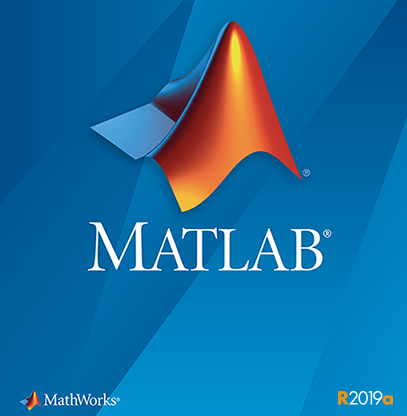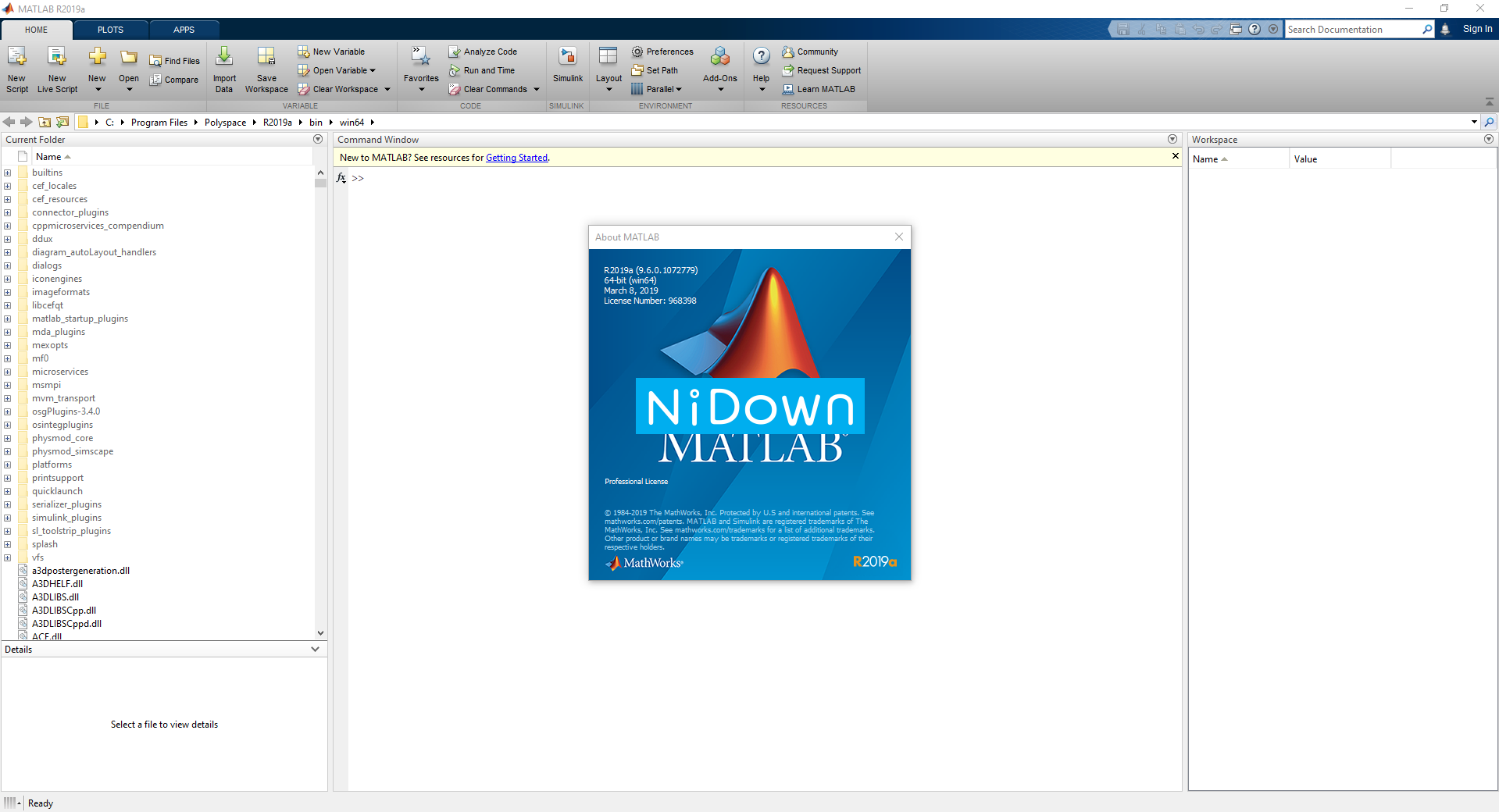# MathWorks MATLAB R2019a Win64 破解版下载 crackMathWorks MATLAB R2019a v9.6.0.1072779 x64 | 破解版 Crack

MATLAB R2019a win 版本正式发布！MATLAB 是美国 MathWorks 公司出品的商业数学软件，用于算法开发、数据可视化、数据分析以及数值计算的高级技术计算语言和交互式环境，主要包括 MATLAB 和 Simulink 两大部分。

MATLAB 是 matrix&laboratory 两个词的组合，意为矩阵工厂（矩阵实验室）。是由美国 mathworks 公司发布的主要面对科学计算、可视化以及交互式程序设计的高科技计算环境。它将数值分析、矩阵计算、科学数据可视化以及非线性动态系统的建模和仿真等诸多强大功能集成在一个易于使用的视窗环境中，为科学研究、工程设计以及必须进行有效数值计算的众多科学领域提供了一种全面的解决方案，并在很大程度上摆脱了传统非交互式程序设计语言（如 C、Fortran）的编辑模式，代表了当今国际科学计算软件的先进水平。

MATLAB 和 Mathematica、Maple 并称为三大数学软件。它在数学类科技应用软件中在数值计算方面首屈一指。MATLAB 可以进行矩阵运算、绘制函数和数据、实现算法、创建用户界面、连接其他编程语言的程序等，主要应用于工程计算、控制设计、信号处理与通讯、图像处理、信号检测、金融建模设计与分析等领域。

MATLAB 的基本数据单位是矩阵，它的指令表达式与数学、工程中常用的形式十分相似，故用 MATLAB 来解算问题要比用 C，FORTRAN 等语言完成相同的事情简捷得多，并且 MATLAB 也吸收了像 Maple 等软件的优点，使 MATLAB 成为一个强大的数学软件。在新的版本中也加入了对 C，FORTRAN，C++，JAVA 的支持。

### 安装激活测试截图